# wave guide

Also found in: Dictionary, Thesaurus, Acronyms, Wikipedia.
Related to wave guide: Rectangular Waveguide
The following article is from The Great Soviet Encyclopedia (1979). It might be outdated or ideologically biased.

## Wave Guide

a dielectric channel, or guiding system, for the propagation of electromagnetic waves. The surface of the channel is an interface between two media. At this interface the dielectric constant ∊, the magnetic permeability μ, and the electrical conductivity σ change abruptly. The surface may be of any shape. Cylindrical wage guides, however, are used most often—especially hollow metallic cylindrical wave guides filled with air or some other gas. The cross section of a metallic wave guide may be, for example, rectangular, circular, U-shaped, or H-shaped (Figure 1). We usually classify as wave guides only channels whose cross sections mathematically are simply connected regions. The propagation of electromagnetic waves in transmission lines with multiply connected cross sections is treated in the theory of long lines. An example of such a transmission line is the two-conductor coaxial line shown in Figure 1,e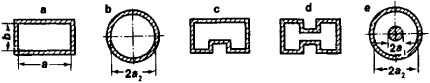Figure 1. (a), (b), (c), and (d) Cross sections of some wave guides; (e) cross section of a coaxial two-conductor line

It can be shown that the wave field propagates inside the wave guide along the guide’s axis; the field is a result of the multiple reflection of the waves from the guide’s inner walls and of the interference of the reflected waves. That propagation occurs in this way accounts for the principal characteristic of wave propagation in wave guides: the propagation of waves in a wave guide is possible only if the transverse dimension of the wave guide is comparable to or greater than the wavelength λ. For example, if λ = 30 cm, the longer dimension a of the cross section of a rectangular wave guide will be 20–25 cm. For this reason, wave guides are used primarily with microwaves.

Wave guides are used as transmission lines in, for example, radar sets for the transmission of energy from the transmitter to the transmitting antenna and from the receiving antenna to the receiver. A microwave transmission line consists of wave-guide sections that differ in shape and in cross-sectional dimensions and of a number of other structures, such as bends and rotary joints (Figure 2). Tapered wave-guide sections, such as the taper in Figure 2, are used to join wave guides of different cross section.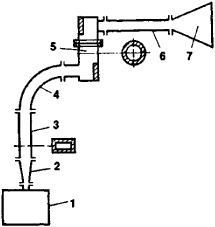Figure 2. Schematic of a microwave wave-guide transmission line: (1) microwave oscillator, (2) taper, (3) and (6) sections of rectangular wave guides, (4) bend, (5) rotary joint, (6) horn antenna

The chief advantage of a metallic wave guide over two-conductor balanced and coaxial lines is that the losses are low at microwave frequencies. There are two reasons for this. First, almost no energy is radiated into the surrounding space. Second, if the outer dimensions of the wave guide and of the, for example, two-conductor line are the same, the wave guide’s surface, on which electric currents flow when waves are propagating, is always greater than the surface of the conductors in the two-conductor line. Since the depth of penetration of the currents is determined by the skine effect, the current density in the wave guide and, consequently, the Joule heat losses are lower than in the line. Four disadvantages of a wave guide can be mentioned. First, there is a lower limit to the frequencies it can pass. Second, the structure required for decimeter or longer waves is unwieldy. Third, a high precision of manufacture and special finishing of the inner surfaces of the walls are required. Fourth, assembly is complicated.

Since the transverse dimensions of a wave guide are comparable to λ, the problems of the propagation and excitation of electromagnetic fields in the wave guide can be solved by integrating Maxwell’s equations for given boundary conditions and field sources. The theory of wave guides deals with the methods for solving these problems. In the case of a rectangular wave guide (Figure 3), for any component f of the electric field E and magnetic field H the theory yields the wave equation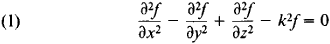where k = 2π/λ = ω/c is the wave number, ω is the oscillation frequency, and c is the speed of light. The solution of this equation for an infinitely long rectangular wave guide reduces to the following expressions for the complex amplitudes of the components of the vectors E and H: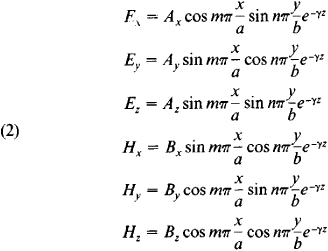Here, a and b are the dimensions of the cross section of the rectangular wave guide, m and n are any positive integers, and Ax, Ay, Az, Bx, By, and Bz are constants determined by the excitation conditions of the wave guide. The propagation constant γ determined by (2) and (1) is given by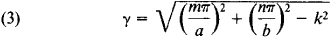The presence of trigonometric factors in (2) indicates the formation of standing waves in directions perpendicular to the walls of the wave guide. The tangential components of the electric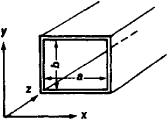Figure 3. Rectangular wave guide

field have nodes at the walls, and the normal components have antinodes. The numbers m and n define the number of half-wavelengths that fit into the dimensions a and b, respectively. The greater the m and n, the more complex the field in the cross section of the wave guide.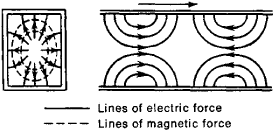Figure 4. Field structure of a TM11 wave In a rectangular wave guide

The wave field in a wave guide is the sum of the fields of an infinite number of wave modes. Three types of wave modes are possible: TE, or H, waves; TM, or E, waves; and TEM waves. The T here stands for “transverse.” Each wave mode has its own field structure. In TE waves, the electric field has only transverse components, but the magnetic field has both longitudinal and transverse components. In TM waves, the magnetic field has only transverse components, and only the electric field has a longitudinal component. TEM waves have no longitudinal field components at all and can exist only in multiply connected wave guides.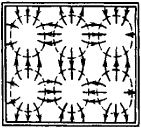Figure 5. Field structure of a TM32 wave in a rectangular wave guide

In the standard notation for wave modes, the m and n appear as subscripts: TMmn and TEmn, or Emn and Hmn. The waves with the lowest possible m and n are called the simplest, or lowest-order, waves. In the case of TM waves, where Hz = 0, the simplest wave is the wave TM11 (Figure 4). TM10 and TM01 waves are not possible because the magnetic field lines must be closed.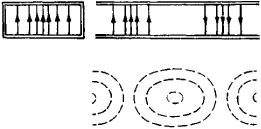Figure 6. Field structure of a TE10 wave in a rectangular wave guide

More complicated waves arise if we increase the transverse dimensions of the wave guide or the frequency so that more than one half-wavelength fits into the dimensions a and b. In this case, the cross section of the wave guide, like a vibrating membrane, is broken up into cells identical in structure to the cross section of a TM11 wave (Figure 5).

TE waves, where Ez = 0, can exist when m = 0 and n 0 or when η ≠ 0 and m ≠ 0. The reason for this is that the electric field lines can be straight lines that originate and terminate on opposite walls of the wave guide (Figures 6 and 7). Higher-order TE waves are composed of “cells” of TE10 and TE11 waves (Figure 8).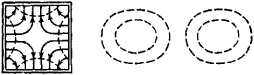Figure 7. Field structure of a TE11 wave in a rectangular wave guide

The factor e-γzdetermines the changes in amplitude and phase of a wave as the wave propagates along the axis of the wave guide. In the absence of losses, γ must be a purely imaginary quantity: γ = iα. Thus,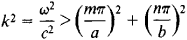This corresponds to the condition for frequency: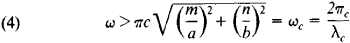This means that a wave guide transmits without attenuation only oscillations of a frequency that is higher than some limiting frequency ωc, which is called the cutoff frequency. To ωc there corresponds the critical, or cutoff, wavelength λc. The smaller the wave-guide dimensions a and b, the higher the cutoff frequency ωc. For a given operating frequency ω, higher-order waves—that is, modes with larger m and n— require larger a and b.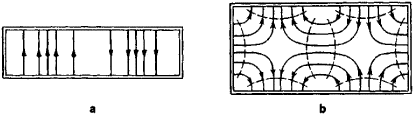Figure 8. Field structures of two types of waves in a rectangular wave guide: (a) TE20 wave, (b) TB21 wave

The wavelength inside the wave guide ∧ is greater than the wavelength in free space: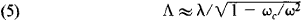The phase velocity of propagation inside the wave guide is equal to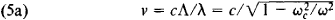that is, the phase velocity is always greater than the speed of light and depends on the frequency. Thus, a wave guide is dispersive. The wider the frequency range of the transmitted signals, the greater the distortion caused by dispersion in the signals.

The attenuation of a wave in a wave guide is described by the real part of the complex propagation constant γ = β + i α. In actual wave guides this attenuation is accounted for by losses in the walls and in the dielectric that fills the wave guide. If ω < ωc in an ideal, or loss-free, wave guide, the electromagnetic field is attenuated without any energy losses, owing to total reflection. The wave-guide dimensions can be chosen so as to permit the wave guide to operate at one of the TE modes. For example, for a rectangular wave guide and the TE10 mode, the choice of a is governed by the condition α > λ > 2a. The dimension a is usually taken as a = 0.72λ cm. Thus, a = 72 mm for λ = 10 cm, and a = 23 mm for λ = 3.2 cm (see Table 1).

The sum of the TE and TM waves in each wave guide forms the complete wave system. This means that propagation in a wave guide is possible only for electromagnetic fields with structures that can be represented as the superposition of TE and TM waves.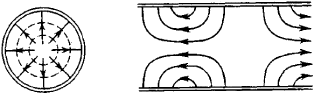Figure 9. Field structure of a TM01 wave in a circular wave guide

For wave guides of circular cross section, the basic equation is not (1) but the Bessel equation, which has solutions in the form of cylindrical functions. In a circular wave guide, it is also possible to choose the diameter so that the wave guide can transmit only one of the first modes (see Table 1). The first mode, however, is not always the most convenient one. For example, because of the axial symmetry of the fields of the TM0Ia and TE01 waves in a circular wave guide (Figures 9 and 10), these waves are used in rotary joints. Figures 11 and 12 show field structures for TM11 and TE11 waves in a circular wave guide. The use of waves with a comparatively small Xc is difficult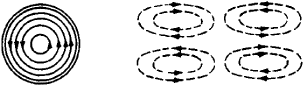Figure 10. Field structure of a TE01 wave in a circular wave guide

because if the propagation conditions for these waves are satisfied, unwanted modes of all other types will also be propagated at the same time.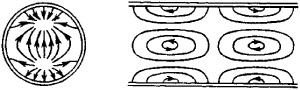Figure 11. Field structure of a TM11 wave in a circular wave guide

The TE01 wave in a circular wave guide has an unusual property: the losses in the walls of the wave guide decrease continuously as λ decreases. This property can be made use of to construct wave-guide communications lines in the millimeter wavelength range with repeater stations spaced 50–60 km apart. Such lines are capable of carrying up to 1,500 telephone channels and 100 television channels. The principal difficulty here consists in providing the necessary field purity of the TE01 wave along the entire line through the elimination of the other wave modes that arise as a result of various discontinuities. In lossy wave guides the concept of a sharp transmission cutoff at ωc

Table 1. Cutoff wavelengths λc for rectangular and circular wave guides
Rectangular wave guideCircular wave guide
Wave mode ........TE10TE20TE10TE11TM01TE21TM11TE01
λc..............2aa2b3.41p2.61p2.06p1.64p1.64p

loses its simple meaning. Lossy wave guides transmit some waves, although weakly, that are beyond the cutoff wavelength (λ > λc) computed for a loss-free wave guide.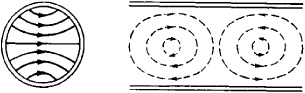Figure 12. Field structure of a TE11 wave in a circular wave guide

Dielectric wave guides can be used for the transmission of centimeter and millimeter waves. In such wave guides the inner surface of a dielectric rod serves as the boundary that guides the wave. Since dielectric wave guides are sensitive to external influences and have additional losses associated with energy leakage beyond the boundaries of the wave guide, practical application is difficult.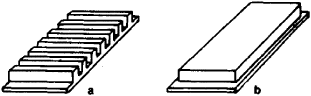Figure 13. Surface-wave wave guides: (a) with ribbed surface, (b) with dielectric coating

In surface-wave wave guides the surface of a metallic strip or a cylindrical conductor is ribbed or is coated with a dielectric (Figure 13). Waves of various modes, for example, TM10, can propagate along such wave guides. The energy of the field is concentrated in the surrounding space. The field radius—that is, the distance at which the field is still appreciable—depends on the width and conductivity of the strip; this radius decreases rapidly as λ decreases. Surface-wave guides exhibit less attenuation than metallic wave guides, are simpler in design, and are capable of transmitting greater power in a wide range of frequencies. The field of the surface wave surrounds the wave guide on the outside, a circumstance responsible for the disadvantages of such wave guides: various discontinuities—such as deformations of the wave guide, supports, joints, and shields—result in radiation, that is, losses of energy. Despite these disadvantages, surface-wave wave guides are used as guiding systems and as radiating elements in decimeter-, centimeter-, and millimeter-wave antennas.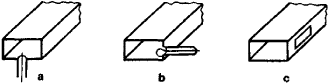Figure 14. Methods of excitation of a TE10 wave: (a) by a probe, (b) by a loop, (c) by an aperture

Three methods of field excitation are used in wave guides: by means of a probe, or linear conductor; by means of a loop; and by means of an aperture, or slot, in the side wall or end face of the wave guide. The probe is located parallel to the lines of electric force. The plane of the loop is perpendicular to the lines of magnetic force. The aperture is cut through the metallic surface in the direction of the lines of magnetic force on this surface. For better coupling, the exciting elements are located in the an-tinodes of the electric or magnetic field (Figure 14).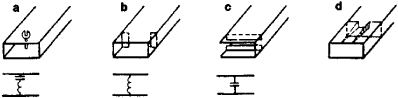Figure 15. Matching devices: (a) reactive probe, (b) inductive iris, (c) capacitive iris, (d) taper

The impedance matching of individual wave-guide sections to each other or to the load can be achieved by means of matching devices (Figure 15) in the form of combinations of probes and inductive or capacitive irises. Tapers are also often used as matching devices. A disadvantage of most matching devices is the small frequency range for which the devices can be used. Matching can generally be achieved in a range of frequencies that is 1–2 percent of ω; 10–20 percent of ω is possible in only a few cases.

The problem of constructing high-power wave guides is of practical importance. The power that a wave guide with cross-sectional dimensions appropriate for the propagation of only first-mode waves can transmit is merely of the order of 3–4 megawatts. If larger dimensions are used for a given wavelength, higher-order modes will propagate through the wave guide.

### REFERENCES

Vvedenskii, B. A., and A. G. Arenberg. Radiovolnovody, part 1. Moscow-Leningrad, 1946.
Kisun’ko, G. V. Elektrodinamikapolykh sistem. Leningrad, 1949.
Vainshtein, L. A. Difraktsiia elektromagnitnykh i zvukovykh voln na ot-krytom kontse volnovoda. Moscow, 1953.
Kaznacheev, Iu. I. Shirokopolosnaia dal’niaia sviaz’ po volnovodam. Moscow, 1959.
Kogan, N. L., B. M. Mashkovtsev, and K. N. Tsibisov. Slozhnye volno-vodnye sislemy. Leningrad, 1963.
Teoriia linii peredachi sverkhvysokikh chastot, parts 1–2. Edited by A. J. Shpuntov. Moscow, 1951. (Translated from English.)
Gurevich, A. G. Polye resonatory i volnovody: Vvedenie ν teoriiu. Moscow, 1952.
Lewin, L. Sovremennaia teoriia volnovodov. Moscow, 1954. (Translated from English.)
Shirman, Ia. D. Radiovolnovody i ob”emnye rezonatory. Moscow, 1959.
Vainshtein, L. A. Elektromagnitnye volny. Moscow, 1957.
Katsenelenbaum, B. Z. Vysokochastolnaia elektrodinamika. Moscow, 1966.
Lebedev, I. V. Tekhnika ipribory SVCh, 2nd ed., vol. 1. Moscow, 1970.
Harvey, A. F. Tekhnika sverkhvysokikh chastot. Moscow, 1968. (Translated from English.)
Fel’dshtein, A. L., et al. Spravochnik po elementam volnovodnoi tekhniki. Moscow, 1967.

I. V. IVANOV

The Great Soviet Encyclopedia, 3rd Edition (1970-1979). © 2010 The Gale Group, Inc. All rights reserved.

## wave guide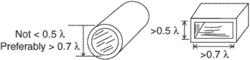In order to efficiently propagate an electromagnetic field, a rectangular waveguide must have sides measuring at least 0.5 wavelength, and preferably more than 0.7 wavelength. A circular wavelength should be at least 0.6 wavelength in diameter, and preferably 0.7 wavelength or more.
An elongated enclosure usually bounded by a cylindrical or rectangular metal tube for propagating energy by means of electromagnetic waves within the enclosure. It is a hollow transmission line or a metal tube through which high-frequency electromagnetic waves are directed. The electromagnetic radiation is conducted in a reverse sense in that radiation travels through the insulator, usually the atmosphere, surrounded by metal along which the waves propagate by multiplying internal reflection. A wave guide must have a minimum dimension that is proportional to the working wavelength. The dimensions of the tube should be greater than half the wavelength of the signal to be used.
References in periodicals archive ?
A typical IO interferometer consists of a high index thin film optical wave guide deposited on a low index substrate.
The components include arrayed wave guides that process up to 40 separate wavelengths of light, a new generation of compact optical amplifiers, a new class of laser pumps and a 10Gb/s (OC-192) optical interface that integrates a laser, laser driver, alarms and a multiplexer in a single unit.
They explain that this situation occurs, for example, in quantum wave guides and for the motion of nuclei in electronic potential surfaces in quantum molecular dynamics.
He begins with "What is electrodynamics?" Subsequent chapters discuss basic aspects, applications, and macroscopic electrostitics; magnetostatics; the Maxwell equations; applications to coils, circuits, and transmission lines; electromagnetic waves; optic laws and diffraction; wave guides and resonators; propagation of radio waves in the ionosphere; the Lagrange formalism for the electromagnetic field; the gauge covariant Schrodinger equation and the Aharono-Bohn effect, among other topics.
Fidelis owns a unique patent pending solar cell technology based on photovoltaic cells with integral light-transmitting wave guides in a ceramic sleeve.
Its range of plant is from an unbelievably small 5 litre project for wave guides to 2000 litre plants for volume production of aircraft parts.
Modular, prefabricated aluminium wave guides transfer microwaves from the transmitter to the applicator and can be quickly connected to fit almost any layout and save floor space.
Up to 512 soil probes or wave guides can be multiplexed and monitored.
The researchers are trying to adapt the technique to devices called "wave guides," which are like fiber-optic sheets.
They look at microwaves in Korea, computers in Singapore, tool-and-dye in Germany, optical wave guides in Japan, even ball-point pens in Rhode Island.
Here, fractal metamaterials are configured as magnifiers and wave guides, producing super-thin flat lenses, bendable conformal lenses, and even edge-oriented lenses, with broad bandwidths.

Site: Follow: Share:
Open / Close